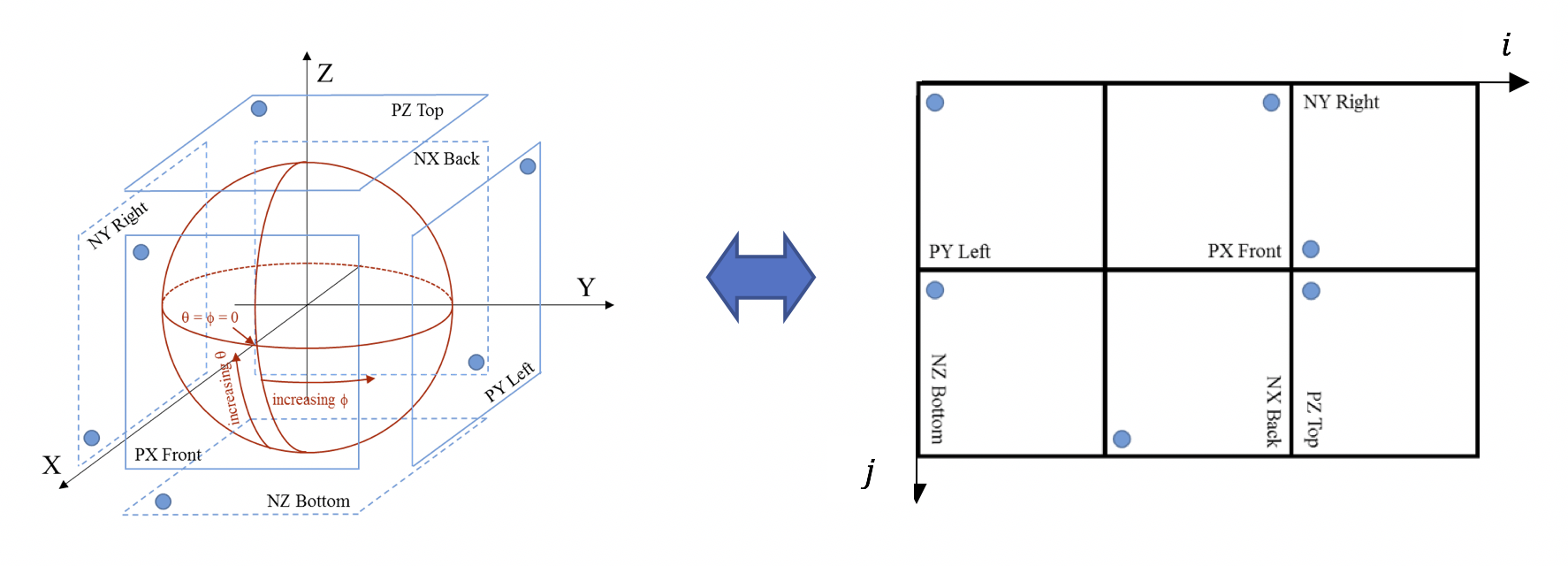## A.2.3.3 Cubemap Projection (CMP)

26.1183GPPRelease 17TSVirtual Reality (VR) profiles for streaming applications

Figure A.3 shows the CMP projection with 6 square faces, labelled as PX, PY, PZ, NX, NY, NZ (with "P" standing for "positive" and "N" standing for "negative"). Table A.2-4 specifies the face index values corresponding to each of the six CMP faces.Figure A.3: Relation of the cube face arrangement of the projected picture to the sphere coordinates

Table A.2-4: Face index of CMP

 Face index Face label Notes 0 PX Front face with positive X axis value 1 NX Back face with negative X axis value 2 PY Left face with positive Y axis value 3 NY Right face with negative Y axis value 4 PZ Top face with positive Z axis value 5 NZ Bottom face with negative Z axis value

The 3D coordinates (X, Y, Z) are derived using following equations:

lw = pictureWidth / 3
lh = pictureHeight / 2
tmpHorVal = i − Floor( i ÷ lw ) * lw
tmpVerVal = j − Floor( j ÷ lh ) * lh
i′ = −( 2 * tmpHorVal ÷ lw ) + 1
j′ = −( 2 * tmpVerVal ÷ lh ) + 1
w = Floor( i ÷ lw )
h = Floor( j ÷ lh )
if( w = = 1 && h = = 0 ) { // PX: positive x front face
X = 1.0
Y = i′
Z = j′
} else if( w = = 1 && h = = 1 ) { // NX: negative x back face
X = −1.0
Y = −j′
Z = −i′
} else if( w = = 2 && h = = 1 ) { // PZ: positive z top face
X = −i′
Y = −j′
Z = 1.0
} else if( w = = 0 && h = = 1 ) { // NZ: negative z bottom face
X = i′
Y = −j′
Z = −1.0
} else if( w = = 0 && h = = 0 ) { // PY: positive y left face
X = −i′
Y = 1.0
Z = j′
} else { // ( w = = 2 && h = = 0 ), NY: negative y right face
X = i′
Y = −1.0
Z = j′
}